International
Tables for
Crystallography
Volume D
Physical properties of crystals
Edited by A. Authier

International Tables for Crystallography (2006). Vol. D, ch. 1.1, p. 32

## Section 1.1.5.2. Other forms of the piezoelectric constants

A. Authiera*

aInstitut de Minéralogie et de la Physique des Milieux Condensés, Bâtiment 7, 140 rue de Lourmel, 75015 Paris, France
Correspondence e-mail: aauthier@wanadoo.fr

#### 1.1.5.2. Other forms of the piezoelectric constants

| top | pdf |

We use here another Gibbs function, the electric Gibbs function,, defined by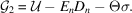Differentiation ofgives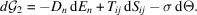It follows that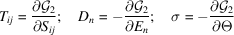and a set of relations analogous to (1.1.5.1):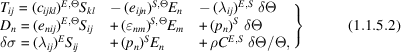where the components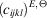are the isothermal elastic stiffnesses at constant field and constant temperature,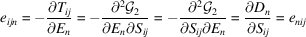are the piezoelectric stress coefficients at constant strain and constant temperature,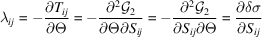are the temperature-stress constants and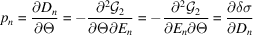are the components of the pyroelectric effect at constant strain.

The relations between these coefficients and the usual coefficients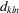are easily obtained:

 (i) At constant temperature and strain: if one puts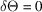and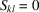in the first equation of (1.1.5.1)and (1.1.5.2), one obtains, respectively,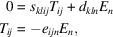from which it follows that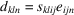at constant temperature and strain. (ii) At constant temperature and stress: if one putsand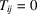, one obtains in a similar way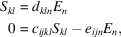from which it follows that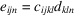at constant temperature and stress.